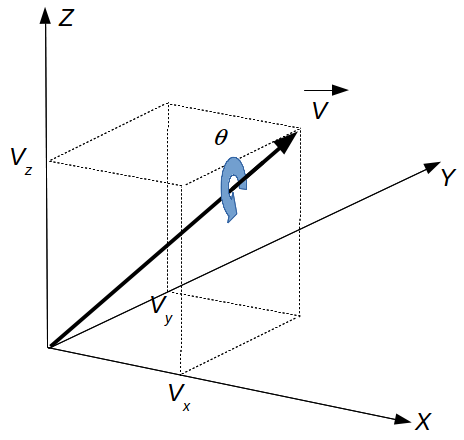Do me a favor, take a few seconds to have a look at my last project.

Thank you, Lulu

# Quaternions and rotations

## Introduction

Quaternion is a generalization of complex numbers with three imaginary numbers ($$i$$, $$j$$ and $$k$$ ). It is a four-dimensional complex number that can be used to represent the orientation of a rigid body or coordinate frame in three-dimensional space. The general definition of a quaternion is given by:

$$Q=a+b.i+c.j+d.k = \left[ \begin{matrix} a && b && c && d \end{matrix} \right]$$

## Representation

Quaternions represents a rotation tranformation in 3D. It can be expressed from Euler angles as on this online visualization. Therefore, the easiest way to represent a quaternion is to imagine the rotation of a given angle around a given vector. The following figure illustrates the rotation of angle $$\theta$$ around vector $$\vec{V}$$ defined by 3 scalars ( $$V_x$$, $$V_y$$ and $$V_z$$) :The quaternion associated to this transformation is given by:

$$Q = \left[ \begin{matrix} q_w && q_x && q_y && q_z \end{matrix} \right]$$

$$Q = \left[ \begin{matrix} cos \frac{\theta}{2} && V_x.sin \frac{\theta}{2} && V_y.sin \frac{\theta}{2} && V_z.sin \frac{\theta}{2} \end{matrix} \right]$$

## Rotation around axes

Based on the previous formula, we can now calculate the quaternion defining a rotation around each axis:

### Rotation around X

$$Q_X=\left[ \begin{matrix} cos \frac{\theta}{2} && sin \frac{\theta}{2} && 0 && 0 \end{matrix} \right]$$

### Rotation around Y

$$Q_Y=\left[ \begin{matrix} cos \frac{\theta}{2} && 0 && sin \frac{\theta}{2} && 0 \end{matrix} \right]$$

### Rotation around Z

$$Q_Z=\left[ \begin{matrix} cos \frac{\theta}{2} && 0 && 0 && sin \frac{\theta}{2} \end{matrix} \right]$$

### General rotation

Let's now assume we want to calculate the coordinates of a given vector $$\vec{v}_A$$ (or point) rotated according to the quaternion $${}^BQ_A$$. The resulting vector $$\vec{v}_B$$ ) can be calculated by the following formula based on the quaternion product and quaternion conjugate.

$$\vec{V}_B = {}^BQ_A \otimes \vec{V}_A \otimes \overline {{}^BQ_A}$$

Note that $$\vec{V}_A$$ and $$\vec{V}_B$$ live in $$\mathbb{R}^4$$ while $$\vec{v}_A$$ and $$\vec{v}_B$$ live in $$\mathbb{R}^3$$. $$\vec{V}_A$$ and $$\vec{V}_B$$ are pure quaternions whose real parts are zero:

$$\vec{V}_A = \begin{bmatrix} 0 \\ \vec{v}_A \end{bmatrix} = \begin{bmatrix} 0 \\ x_A \\ y_A \\ z_A \end{bmatrix}$$

$$\vec{V}_B = \begin{bmatrix} 0 \\ \vec{v}_B \end{bmatrix} = \begin{bmatrix} 0 \\ x_B \\ y_B \\ z_B \end{bmatrix}$$

Another way to visualize the previous result is to consider that $$\vec{Q_{V_A}}$$ and $$\vec{Q_{V_B}}$$ are the same vector described in frame $$A$$ and frame $$B$$ respectively. The quaternion $${}^BQ_A$$ represents the transformation from frame $$A$$ and frame $$B$$.

## ExampleThis simple example shows the rotation of an ellipse defined in the plane { $$\vec{X}$$ , $$\vec{Y}$$ }. The green and red points are respectively the initial and final position of the ellipses. The black dash-dotted line is the rotation axis. The rotation angle is $$\frac {\pi}{4}$$ and the rotation vector is given by:

$$V_R = \begin{bmatrix} 1 \\ 1 \\ 0 \end{bmatrix}$$

## Download

Files for the previous example can be downloaded here:

ellipse_rotation.zip

Credit: based on the Madgwick's quaternion library for Matlab.

## See also

Last update : 04/13/2019